# High School Math : How to find out if lines are perpendicular

## Example Questions

### Example Question #1 : How To Find Out If Lines Are Perpendicular

Which of the following are perpendicular to the line with the formula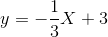?

I.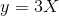II.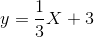III.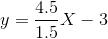III

I and III

II

II and III

I

I and III

Explanation:

The slope of a perpendicular line is equal to the negative reciprocal of the original line.  This means that the slope of our perpendicular line must be 3.  We can also note that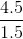is also equal to 3, so both of these slopes are correct.  The y-intercept does not matter, as the slope is the only thing that determines the slant of the line.  Therefore, numerals I and III are both correct.

### Example Question #1 : How To Find Out If Lines Are Perpendicular

Which of the following lines is perpendicular to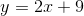?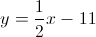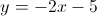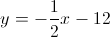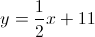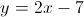Explanation:

In order for two lines to be perpendicular to each other, their slopes must be opposites and reciprocals of each other, meaning the fraction must be flipped upside down and the signs must be changed. In this situation, the original equation had a slope of, so the perpendicular slope must be### Example Question #2 : How To Find Out If Lines Are Perpendicular

Which of the following lines will be perpendicular to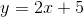?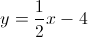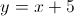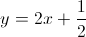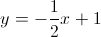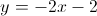Two lines are perpendicular if they have opposite reciprocal slopes. When a line is in standardform, theis the slope. A perpendicular line will have a slope of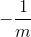.
The slope of our given line is. Therefore we want a slope of. The only line with the correct slope is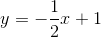.